• 论文研究-区间数判断矩阵的一致性检验及排序方法 .pdf,
• 论文研究-语言判断矩阵的满意一致性检验方法.pdf, 分析了语言判断矩阵具有满意一致性定义的合理性, 定义了一个满意的一致性指标; 给出了满意一致性指标的计算方法, 通过...
• 论文研究-可能满意度与判断矩阵的一致性检验及改进.pdf, 对判断矩阵一致性的检验与改进是层次分析法中的重要问题.针对现有检验与调整方法存在的不足,将"可能满意度"的...
• 论文研究-一种检验判断矩阵次序一致性的实用方法.pdf, 论述了在层次分析法研究与应用中保持两两比较判断矩阵次序一致性的必要性, 并给出一种检验次序一致性的实用方法...
• 层次分析法中判断矩阵的填写方法一致性检验的步骤、以及根据判断矩阵计算权重的方法

目录：

准则层判断矩阵怎么填写
方案层判断矩阵怎么填写
关于判断矩阵和一致矩阵的知识点补充
一致性检验的步骤
怎样通过判断矩阵去计算权重（三种方法），及相应的代码示例

准则层判断矩阵的填写：
填写准则层判断矩阵的目的是确定各准则（指标）所占的比重，填写好层次分析表的指标权重列，例如在选择最佳旅游地问题的指标景色、花费、居住、饮食、交通各自占比是多少，后续可以通过这些指标占比计算出每一个可选方案的总分。填表的方法是依据标度表，两两比较指标的重要程度，只需要比较10次就可以完成准则层判断矩阵的填写

方案层判断矩阵的填写
填写方案层判断矩阵的目的是给出，对于某一特定指标，它在各个可选方案的具体得分是多少，也就是给出层次分析表的每一横行的数据。方法是依据标度表，填写好判断矩阵。有几个评价指标，就需要填多少此方案层判断矩阵。知识点补充：
判断矩阵（正互反矩阵）

首先判断矩阵一定是一个方阵
判断矩阵每一个数据 Aij表示与指标 j相比 i的重要程度
当 i=j 时，两个指标相同，因此同等重要，记为1，因此判断矩阵的对角线元素为1
每一个元素均大于零，且 Aij * Aji=1

在层次分析法中，我们构造的矩阵的均为判断矩阵
一致矩阵

矩阵首先满足判断矩阵的所有特点
若判断矩阵满足 Aij * Ajk = Aik,直观的看就是矩阵的各行（各列）成倍数关系

注意点：在使用判断矩阵求权重之前，必须对其进行一致性检验

一致性检验的步骤：
第一步：计算一致性指标CI
$CI\,\,=\,\,\frac{\lambda _{\max}-n}{n-1}$
第二步：查找对应的平均随机一致性指标RI第三步：计算一致性比例CR
$CR\,\,=\,\,\frac{CI}{RI}$
判断：如果CR<0.1,则可认为判断举证的一致性可以接受；否则需要对判断矩阵进行修改
一致性检验的MATLAB代码如下：
disp('请输入判断矩阵A')
A=input('A=');
[n,n] = size(A);
[V,D] = eig(A);%求出矩阵A的特征值和特征向量
Max_eig = max(max(D));%找到矩阵A的最大特征值
% 下面是计算一致性比例CR的环节 %
CI = (Max_eig - n) / (n-1);
RI=[0 0.0001 0.52 0.89 1.12 1.26 1.36 1.41 1.46 1.49 1.52 1.54 1.56 1.58 1.59];
%注意哦，这里的RI最多支持 n = 15
% 这里n=2时，一定是一致矩阵，所以CI = 0，我们为了避免分母为0，将这里的第二个元素改为了很接近0的正数
CR=CI/RI(n);
disp('一致性指标CI=');disp(CI);
disp('一致性比例CR=');disp(CR);
if CR<0.10
disp('因为CR<0.10，所以该判断矩阵A的一致性可以接受!');
else
disp('注意：CR >= 0.10，因此该判断矩阵A需要进行修改!');
end



通过判断矩阵求权重
方法一、算数平均法求权重
第一步：将判断矩阵按照列归一化（每一个元素除以器所在列的和）
第二步：将归一化的各列相加（按行求和）
第三步：将相加后得到的向量中的每个元素除以n即可得到权重向量
具体数学表达：
假设判断矩阵为下面这个矩阵A：
$A=\left[ \begin{matrix} a_{11}& a_{12}& \cdots& a_{1n}\\ a_{21}& a_{22}& \cdots& a_{2n}\\ \vdots& \vdots& \ddots& \vdots\\ a_{n1}& a_{n2}& \cdots& a_{nn}\\ \end{matrix} \right]$
那么算数平均法求得的权重向量为：
$w_i=\frac{1}{n}\sum_{j=1}^n{\frac{a_{ij}}{\sum_{k=1}^n{a_{kj}}}}\,\,$
MATLAB代码如下：
disp('请输入判断矩阵A')
A=input('A=');
[n,n] = size(A);

Sum_A = sum(A);   %sum函数默认是对矩阵的每一列进行累加，即按行求和
SUM_A = repmat(Sum_A,n,1);    %将Sum_A这个行向量，重复n行，重复一列
Stand_A = A ./ SUM_A;         %将矩阵A归一化，即每一个元素除以其所在列的和

disp('算术平均法求权重的结果为：');
disp(sum(Stand_A,2)./n)      %把归一化的矩阵的每一行累加，然后除以n,得到权重

方法二、几何平均法求权重
第一步：将A元素按照行相乘得到一个新的列向量
第二步：将新的列向量的每个分量开n次方
第三步：对该列向量进行归一化即可得到权重向量
假设判断矩阵为下面这个矩阵A：
$A=\left[ \begin{matrix} a_{11}& a_{12}& \cdots& a_{1n}\\ a_{21}& a_{22}& \cdots& a_{2n}\\ \vdots& \vdots& \ddots& \vdots\\ a_{n1}& a_{n2}& \cdots& a_{nn}\\ \end{matrix} \right]$
那么几何平均法求得的权重向量为：
$w_i=\frac{\left( \prod_{j=1}^n{a_{ij}} \right) ^{\frac{1}{n}}}{\sum_{k=1}^n{\left( \prod_{j=1}^n{a_{kj}} \right) ^{\frac{1}{n}}}},\left( i=1,2,…\text{，}n \right)$
注意：每一种方法求得的权重和应该为1，由于四舍五入导致的误差可以忽略，一般结果保留四位小数
MATLAB代码如下：
disp('请输入判断矩阵A')
A=input('A=');
[n,n] = size(A);  %获得矩阵A的行和列的大小

Prduct_A = prod(A,2);       %把矩阵A的每一行累乘，即按照列累乘
Prduct_n_A = Prduct_A .^ (1/n);   %将新的列向量的每个分量开n次方
disp('几何平均法求权重的结果为：');
disp(Prduct_n_A ./ sum(Prduct_n_A))  %对该列向量进行归一化即可得到权重向量

方法三、特征值法求权重（常用）
知识点提醒：一致矩阵有一个特征值为n,其余特征值均为0，并且当矩阵的特征值为n时，其对应的特征向量为
$k\left[ \frac{1}{a_{11}},\frac{1}{a_{12}},…,\frac{1}{a_{1n}} \right] ^T,\left( k\ne 0 \right)$
第一步：求出矩阵A的最大特征值和以及其对应的特征向量
第二步：对求出的特征向量进行归一化即可得到所求的权重
MATLAB代码如下：
disp('请输入判断矩阵A')
A=input('A=');

%求矩阵A的全部特征值，构成对角阵D，求A的特征向量构成V的列向量（V的每一列都是D中与之相同列的特征值的特征向量）
[V,D] = eig(A);
Max_eig = max(max(D));   %求出矩阵A的最大的特征值
[r,c]=find(D == Max_eig , 1);  %返回最大特征值所在的行和列，其中C记录所在列
disp('特征值法求权重的结果为：');
disp( V(:,c) ./ sum(V(:,c)) )  %对最大特征值对应的特征向量进行归一化处理

友情提示：在比赛当中，建议三种方法全部列出来，但仅适用特征值法求得的权重结果进行计算

更多有关与层次分析法的经典获奖论文，以及建模论文模板等资料关注公众号，回复，“层次分析法”，即可免费领取！！！展开全文• disp('请输入判断矩阵A') A=input('A='); [n,n] = size(A) %方法1： 算术平均法 Sum_A = sum(A); SUM_A = repmat(Sum_A,n,1); Stand_A = A ./ SUM_A; Stand_A = A ./ Sum_A; % 这样也可以 disp('算术平均法求权重...
Matlab源程序代码如下：
clc
clear
disp('请输入判断矩阵A')
A=input('A=');
[n,n] = size(A)
%方法1： 算术平均法
Sum_A = sum(A);
SUM_A = repmat(Sum_A,n,1);
Stand_A = A ./ SUM_A;
Stand_A = A ./ Sum_A; % 这样也可以的
disp('算术平均法求权重的结果为：');
disp(sum(Stand_A,2)./n)
%方法2： 几何平均法
Prduct_A = prod(A,2);
Prduct_n_A = Prduct_A .^ (1/n);
disp('几何平均法求权重的结果为：');
disp(Prduct_n_A ./ sum(Prduct_n_A))
%方法3： 特征值法求权重
[V,D] = eig(A);
Max_eig = max(max(D))
[r,c]=find(D == Max_eig , 1);
disp('特征值法求权重的结果为：');
disp( V(:,c) ./ sum(V(:,c)) )
%计算一致性比例CR
CI = (Max_eig - n)/(n-1);
RI=[0 0.0001 0.52 0.89 1.12 1.26 1.36 1.41 1.46 1.49 1.52 1.54 1.56 1.58
1.59];
% 这里n=2时，一定是一致矩阵，所以CI = 0，为了避免分母为0，将这里的第二个元素改为了很接近0的正数
CR=CI/RI(n);
disp('一致性指标CI=');disp(CI);
disp('一致性比例CR=');disp(CR);
if CR<0.10
disp('因为CR<0.10，所以该判断矩阵A的一致性可以接受!');
else
disp('注意：CR >=
0.10，因此该判断矩阵A需要进行修改!');
end


展开全文源程序代码
• 为了方便检验语言判断矩阵的一致性,通过引入导出矩阵的概念将语言判断矩阵转化为数量矩阵,并提出了语言判断矩阵完全一致性、满意一致性的简便的判定方法;针对基于语言判断矩阵的方案排序问题,根据导出矩阵的性质通过...
• 论文研究-互补判断矩阵的两种排序方法——权的最小平方法及特征向量法...而且 ,基于转换矩阵 ,提出了互补判断矩阵排序的特征向量法 (CEM) ,给出了其相应的迭代算法及互补判断矩阵的一致性检验方法 .最后进行了算例分析.
• 通过"标度变换"把不具"可加性"比例标度转化为评分标度,利用评分标度"可加性"可计算比较对象得分、并按得分率对比较对象排序。与已有单准则排序方法的实质性区别是,勿需进行一致性检验
• 论文研究-广义判断下AHP(GJAHP)的一致性检验与可接受性的进一步探讨.pdf, 广义判断下的AHP(GJAHP)是在单准则下通过构造广义判断矩阵的数学模型而建立的一种广义AHP.本文...
• 一致性检验、修正和权值排序作为一个整体处理,属于全局分析方法.采用遗传算法优化建立的一致性指标系数函数,引入小生境技术以保证在待优化参数较多时仍能找到最优解.分析...
• 按照Saaty的观点, 当一致性比例CR>0.1时, 则认为判断矩阵的一致性差。文献对此做过研究。本文先给出一个定理, 再由此导出判断矩阵的修正方法, 从而使得逻辑性更强。最后, 本文将给出检验修正效果的两个判据。
• 论文研究-群组判断矩阵排序中广义最小偏差方法.pdf, ...鉴于不同专家所给判断矩阵质量上差异, 广义最小偏差排序方法对群组判断矩阵进行不同程度加权处理, 并进行群组一致性检验
• else disp('此矩阵的一致性验证失败，请重新进行评分!'); end disp('请输入方案层各因素对准则层各因素权重的成对比较阵'); for i=1:n disp('请输入第');disp(i);disp('个准则层因素的判断矩阵B');disp(i);
• 层次分析法理论部分评价类模型——层次分析法，一致性检验学习笔记（一） 层次分析法方法总结： 第一步：分析系统中各因素之间关系，建立系统递阶层次结构 第二步：对于同一层次各元素关于上一层次中某...

前一篇讲述了层次分析的原理，例题讲解，接下来是matlab代码实现

层次分析法理论部分评价类模型——层次分析法，一致性检验学习笔记（一）

层次分析法方法总结：

第一步：分析系统中各因素之间的关系，建立系统的递阶层次结构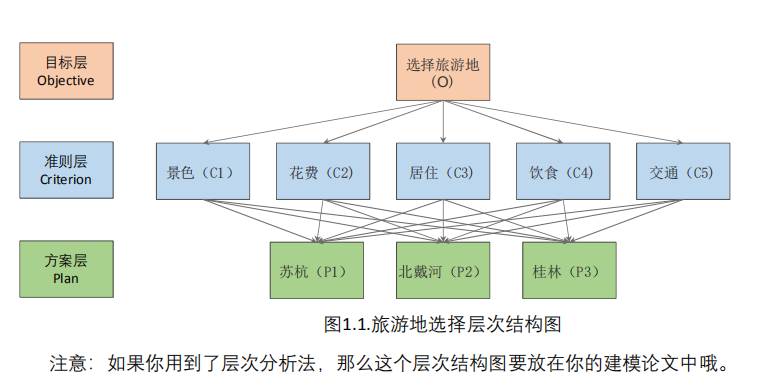第二步：对于同一层次的各元素关于上一层次中某一准则的重要性进行两两比较，构造两两比较矩阵（判断矩阵）。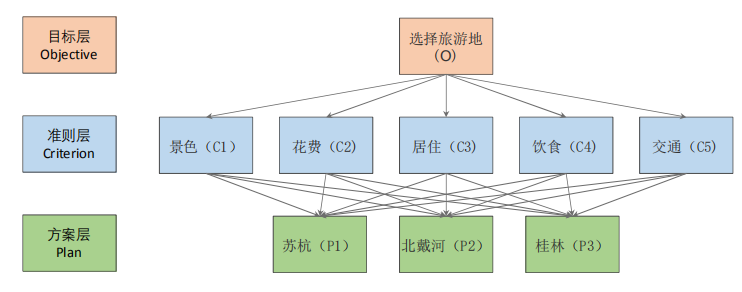1.构建准则层判断矩阵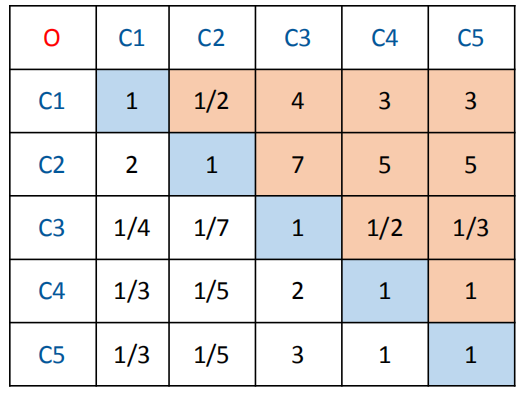2.准则层—方案层的判断矩阵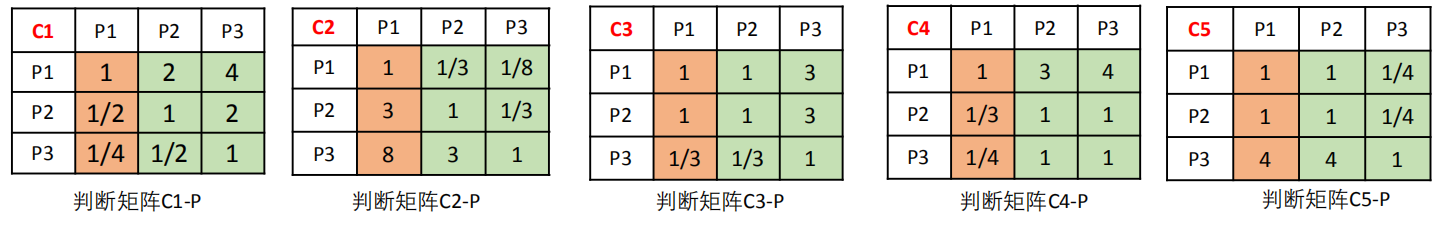第三步： 由判断矩阵计算被比较元素对于该准则的相对权重， 并进行一致性检验（检验通过权重才能用）

三种方法计算权重：

（1）算术平均法（2）几何平均法（3）特征值法

第四步：计算各层元素对系统目标的合成权重，并进行排序。

代码：

以解决景色、花费、居住、饮食、交通为例的判断矩阵的权重为例子：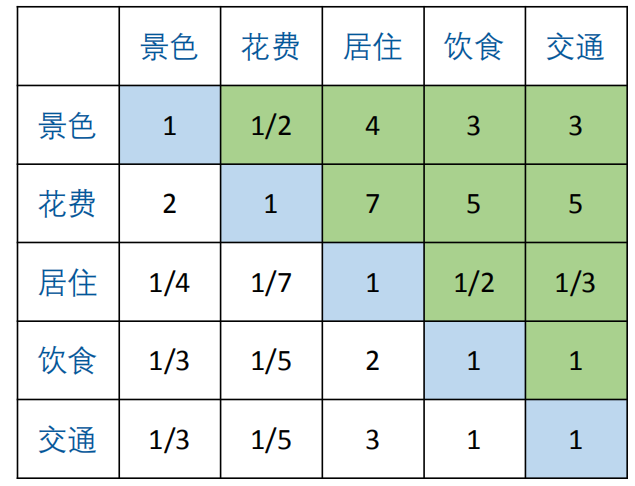新建一个后缀名为.m文件，将代码复制进去，在工作区新建变量并命名为A，填写如下信息

当然也可以通过键盘输入，参考注释语句。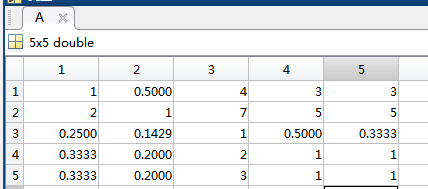具体实现代码如下

%%输入判断矩阵
% clear;
% clc;
%A=input('请输入判断矩阵:')
[n,n]=size(A);%取矩阵列数n
%%
%判断矩阵一致性检验
clc;
[V,D]=eig(A);%求特征值和特征向量，V存放特征向量，D存放特征值
Max_eig=max(max(D));%取D中最大特征值
CI=(Max_eig-n)/(n-1);%计算一致性指标CI
RI=[0 0 0.52 0.89 1.12 1.26 1.36 1.41 1.46 1.49 1.52 1.54 1.56 1.58 1.59];%存放平均随机一致性指标
CR=CI/RI(n);%计算一致比例
% 如果CR < 0.1, 则可认为判断矩阵的一致性可以接受；否则需要对判断矩阵进行修正。
disp('一致性指标CI=');disp(CI);
disp('一致性比例CR=');disp(CR);
if CR<0.10
disp('因为CR < 0.10，所以该判断矩阵A的一致性可以接受!');
else
disp('注意：CR >= 0.10，因此该判断矩阵A需要进行修改!');
end
%%
%方法1：算术平均法求权重
sum_A=sum(A);%对矩阵所在列作和
SUM_A=repmat(sum_A,n,1);%将sum_A看做一个整体，重复n*1块
stand_A=A./SUM_A;%A和SUM_A矩阵对应行相除；即将判断矩阵按照列归一化
disp('算术平均法求权重的结果为：');
disp(sum(stand_A,2) / n)
%%
%方法2：几何平均法求权重
Prduct_A = prod(A,2);%将A的元素按照行相乘得到一个新的列向量
% prod函数和sum函数类似，一个用于乘，一个用于加  dim = 2 维度是行
Prduct_n_A = Prduct_A .^ (1/n);% 这里对每个元素进行乘方操作，这里是开n次方，所以我们等价求1/n次方
disp('几何平均法求权重的结果为：');
disp(Prduct_n_A ./ sum(Prduct_n_A))
%%
%方法3：特征值法求权重
[r,c] = find(D == Max_eig , 1);% 找到D中第一个与最大特征值相等的元素的位置，记录它的行和列。
disp('特征值法求权重的结果为：');
disp( V(:,c) ./ sum(V(:,c)) )%对求出的特征向量进行归一化即可得到我们的权重

实验结果

存储完矩阵A后，点击运行输出结果为：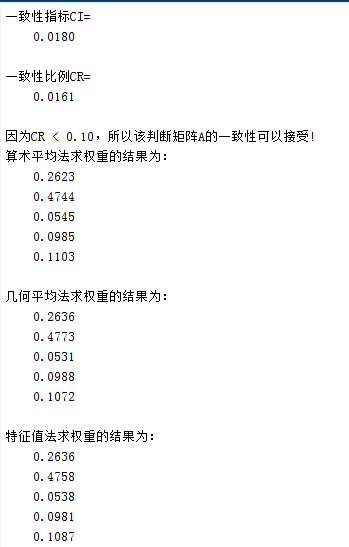清除工作区间后，输入其他的判断矩阵。你学会了吗。

展开全文层次分析法 matlab 评价类模型
• Summary层次分析法在...如何构造判断矩阵以便能一次性通过一致性检验。通晓了如何构造判断矩阵以及熟练掌握yaahp层次分析法软件，相信层次分析不再是你建模、科研路上拦路虎。如果想获得yaahp层次分析法软件（软...
Summary层次分析法在最近的数据咨询案例中经常遇到，其实它是一个很简单有方法可循的常用多目标规划方法。在本文中你将了解到：层次分析法的用途；层次分析模型构造过程；不需要手算和编程的yaahp层次分析法软件介绍；如何构造判断矩阵以便能一次性通过一致性检验。通晓了如何构造判断矩阵以及熟练掌握yaahp层次分析法软件，相信层次分析不再是你建模、科研路上的拦路虎。如果想获得yaahp层次分析法软件（软件与详细使用教程）以及“数学模型（姜启源）pdf电子书（层次分析法在P249，可以帮助深入了解层次分析）”，请将本文转发到朋友圈或一个群（QQ或微信），然后截图发给微信公众号后台（统计程序狗），顺便留下您的邮箱即可哦~引言多目标决策即具有多种选择的目标决策问题。例如，挑选男朋友就是一个多目标决策问题。不同的人对如何找一个理想的男朋友的标准是不同的，有的人更看重相貌，有的人更看重经济条件。但是，许多女孩经常面对的情况是“长得丑的男生家庭条件好“，”长得好看的男生能力又有点欠缺”。因此，在做出选择的时候，我们都会不同程度的因迁就一些因素从而换取自己比较看重的因素，那么当有几个备选男朋友出现而你又难以做出选择时，如何根据自己的喜好，使用可以量化的方法“综合”地选出一个最理想的男朋友呢？这就是多目标决策问题，而层次分析法就是解决这类问题的一个非常重要的手段。因此有诗云：层次选的妙，脱单会更早；分析做的好，爱情更加牢。层次分析法介绍层次分析法的基本思想是把复杂问题分解为若干层次，在每一层次通过两两对比得出判断矩阵、然后计算各因素的权重，接着由低到高地层层分析计算，最后得出各方案对总目标的权重，权重最大的方案即为最优方案。如图1所示，“选一个男朋友”是目标层，目标层一般只有一个；中间的绿色框是准则层和指标层，即我们要考虑的因素；最后蓝色框是方案层，我们的目的是在准则层和指标层下，综合得出相对于目标层而言最优的一个方案。何为最优，层次分析法有一套数学计算方法，最后方案层的每一个方案都会对应一个权重，权重最大者即最优。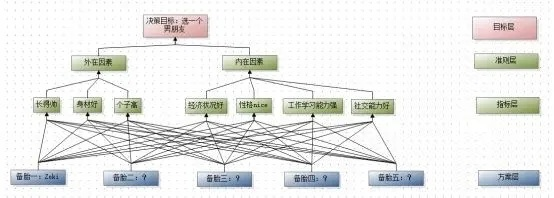图1判断矩阵层次分析的第一步是要对准则层、指标层和方案层的因素进行两两比较从而建立判断矩阵，所谓判断矩阵即每一层的几个因素相对于上一层某一个因素的相对重要性。比如对于外在因素，你觉得“长得帅”、“身材好”以及“个子高”的相对重要性是怎样的？比如有的人认为：长得帅比身材好重要，长得帅比个子高重要，身材好比个子高重要。但是重要的程度又怎样界定呢？可以参考下表：表1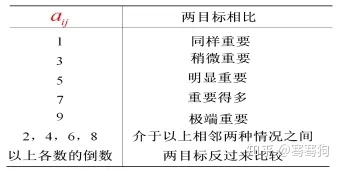其中，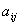表示判断矩阵中第i行第j列的元素，也是第i行个因素比第j列个因素的相对重要性，自然的如果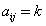，那么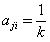。       由表1，我们可以进一步作出这样的判断：在外在因素中，“长得帅”比“身材好”明显重要，长得帅比个子高极端重要，身材好比个子高稍微重要，那么判断矩阵就是：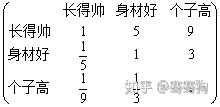判断矩阵的建立是为了得出“长得帅、身材好、个子高”对于决策所占的权重，而权重的得出是用判断矩阵的特征向量表示的。特征向量的涵义即为相对于外在因素，“长得帅”、“身材好以及“个子高”的对于作出决策的重要程度，我们计算出的结果为(0.7482, 0.1804, 0.0714)，所以长得帅、身材好、个子高对于外在因素的重要性依次为0.7482，0.1804，0.0714，像这样，依次构造每一层相对于上一层某因素的判断矩阵并计算权重。然而，我们的目标是做出决策，因此还需要建立目标层“选一个男朋友”与方案层“各备胎”之间的联系。这就需要计算出合成权重，合成权重即为在考虑所有内外在因素的前提下，各备胎的权重比较结果，权重最大者即是最佳男友。yaahp层次分析法软件关于方案层对于目标层的合成权重以及判断矩阵的特征向量计算过程十分繁琐，这里我们给大家介绍一个非常容易操作的层次分析软件（yaahp层次分析法软件），这个软件可以帮我们免去层次分析法复杂的计算过程，直接得出最佳决策。第一步：运行软件。打开软件后，主要分为三个部分，分别是“层次结构模型”，“判断矩阵”以及“计算结果”三个主要界面。第二步：在“层次结构模型”界面中，通过工具栏进行层次结构目标层、准则层、指标层以及方案层的绘制；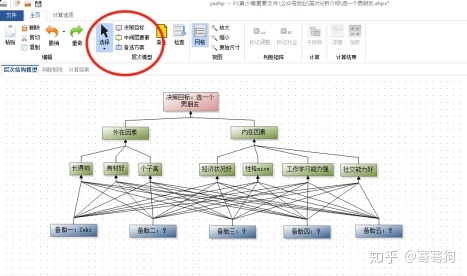第三步：判断矩阵的输入进入“判断矩阵”界面（椭圆1）。在椭圆2处选择对哪个因素设计判断矩阵。点击椭圆3，设计“长得好”相对于“身材好”的重要性（滑动椭圆4中的蓝色光柱）。在椭圆5中直接会出现一致性检验结果，如果一致性检验不通过还得继续调整判断矩阵。依次输入完所有的判断矩阵。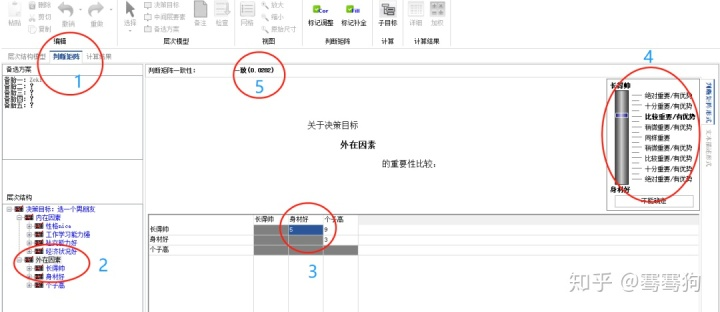第四步：进入“计算结果”界面（椭圆1）。即可以看出各备胎的合成权重（椭圆2）。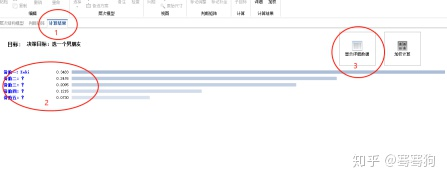点击上图中详细结果（椭圆3），即可见所有计算过程当中的结果(下图)。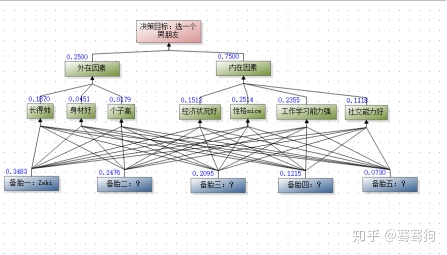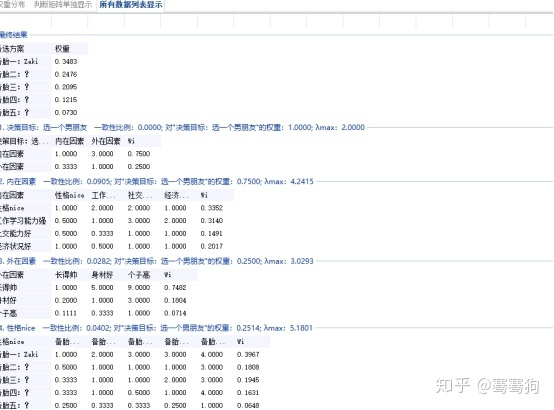没错，最后我们就完成了层次分析，从中可以看出，综合内外在因素，从各个方面综合考虑，最优秀的还是Zeki（自恋的家伙）！！！当然，点击如下计算选项，有更多的计算功能，还有待大家去开发哦！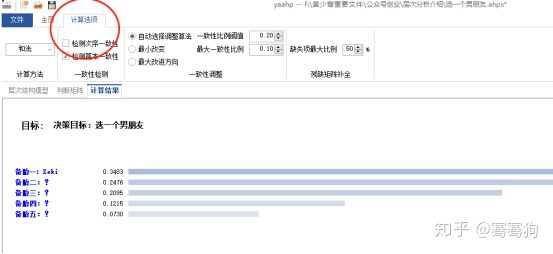关注请微信搜索：统计程序狗（微信号：statistical_dog）
展开全文• 论文研究- 平均随机一致性指标R·I的新探讨.pdf, 一、前言 迄今为止,在AHP一致性检验中,人们普遍采用Saaty推荐的方法,即对于判断矩阵A,当一致性指标μ×R·I时,认为A有满意的一致性,否则,将认为A的一致性不够,须对...
• 论文研究-基于未确知三值判断的...给出了两两比较判断值为未确知三值互反标度时判断矩阵的构造方法,并通过将这种互反判断矩阵转化为属性判断矩阵,给出了其一致性检验方法及权系数的简明计算公式.最后给出了算例分析.
• 在对土地集约利用评价过程中，存在着评价专家选择问题以及AHP打分...利用改进的判断矩阵生成算法，对专家的判断矩阵进行一致性检验，解决可能存在成对比较项目不一致问题，为构建评价指标体系提供有效支持。
• 基本过程是:对所分析的问题建立层次模型,然后将一个层次的各因素相对于上一层次的各准则(或目标)进行两两比较判断,构造判断矩阵,通过对判断矩阵的计算,进行层次单排序和一致性检验,最后进行层次总排序,得
• 相对现有研究贡献在于：关注多方案情景多评价对象、多决策目标和多要素集合特征，依据整体决策信息判定策略和成对要素比较信息序列转换策略，创造性提出初始判断信息整体判断偏好一致性检验方法和多轮次非一致...
• 方法仍具有较强的主观性，判断/比较矩阵的构造在一定程度上是拍脑门决定的，一致性检验只是检验拍脑门有没有自相矛盾得太离谱。 相关的理论参考可见：wiki百科 二、代码实现 需要借助Python的numpy矩阵运算包，...
• 为处理 AHP中判断矩阵的一致性问题 ,直接从判断矩阵的定义出发 ,提出用加速遗传算法同时计算 AHP中各要素的排序权值和检验判断矩阵一致性的新方法 (AGA-AHP) ....
• 3.由判断矩阵计算被比较元素对于该准则相对权重，并进行一致性检验（检验通过权重才能用）。 4.填充权重矩阵。 二、模型 2. 对于同一层次个元素关于上一层次中某一准则重要性两两比较，构造两两比较矩阵...
• 一、层次分析法原理层次分析法...该方法仍具有较强的主观性，判断/比较矩阵的构造在一定程度上是拍脑门决定的，一致性检验只是检验拍脑门有没有自相矛盾得太离谱。相关的理论参考可见：wiki百科二、代码实现...
• 研究了层次分析法(AHP) ... 然后, 提出了层次分析法中简化超传递近似法及群决策几何平均超传递近似法, 该方法不需要一致性检验, 同时又保持了专家原始意见; 最后, 通过一个实例验证了该方法的有效性和实用性.</p>